# How to Perform Arithmetic Operation using Switch Case in PHP through HTML form ?

• Last Updated : 05 Jun, 2021

We are going to perform the basic arithmetic operations like- addition, subtraction, multiplication, and division using PHP. We are using HTML form to take the input values and choose an option to perform particular operation using Switch Case.

Arithmetic Operations are used to perform operations like addition, subtraction etc. on the values. To perform arithmetic operations on the data we need at least two values.

• Addition: It performs the sum of given numbers.
• Subtraction: It performs the difference of given numbers.
• Multiplication: It performs the multiplication of given numbers.
• Division: It performs the division of given numbers.

Example:

```Addition = val + val2 + ... + valn
Example: add = 4 + 4 = 8

Subtraction = val - val2 - ... - valn
Example: sub = 4 - 4 = 0

Multiplication = val1 * val2 * ... * valn
Example: mul = 4 * 4 = 16

Division = val1 / val2
Example: mul = 4 / 4 = 1```

Program 1:

## PHP

 ``

Output:

```Sum: 45
Diff: -15
Mul: 450
Div: 2```

Using Switch Case: The switch statement is used to perform different actions based on different conditions.

Syntax:

```switch (n) {
case label1:
code to be executed if n=label1;
break;
case label2:
code to be executed if n=label2;
break;
case label3:
code to be executed if n=label3;
break;

. . .

case labeln:
code to be executed if n=labellast;
break;
default:
code to be executed if n is different from all labels;
}```

Execution Steps:

• Start XAMPP Server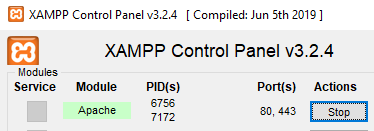• Open Notepad and type the below code and save the folder in path given in image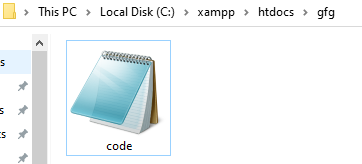Program 2:

## PHP

 ```````    ``GFG``` `
``    ``

``        ``ARITHMETIC OPERATIONS DEMO USING``        ``SWITCH CASE IN PHP``    ``

` `    ``

``    ``

Option-2 = Subtraction

``    ``

Option-3 = Multiplication

``    ``

Option-4 = Division

``    ` `    ``
``        ````            ``
``                ``
``                ``
``                ``
``                ``
``                ````                ````            ``` `            ````            ````            ````            ``` `            ````                ````                ````            ``` `            ````                ````            ````        ````    ````` ``````

Output: Open Web Browser and type localhost/gfg/code.php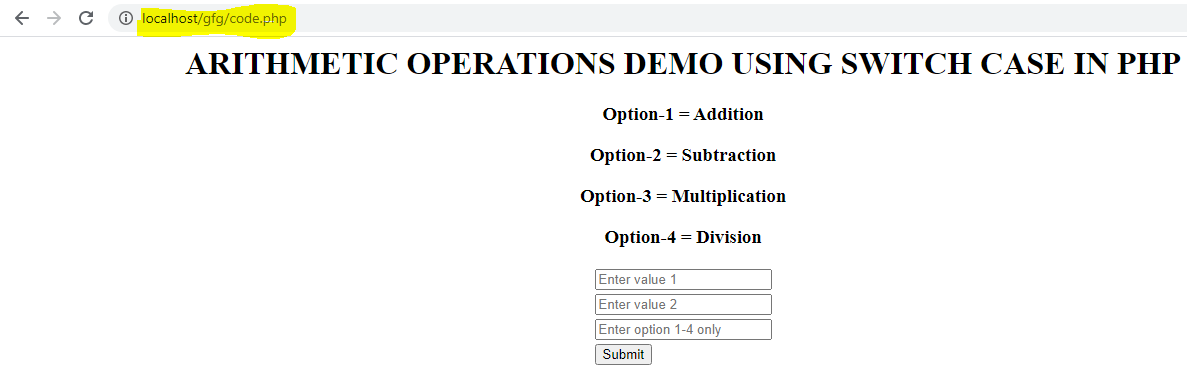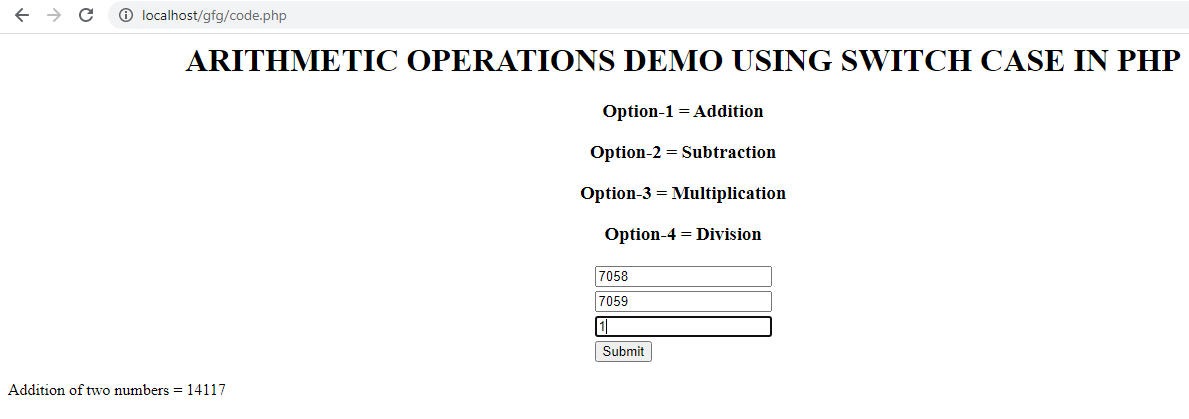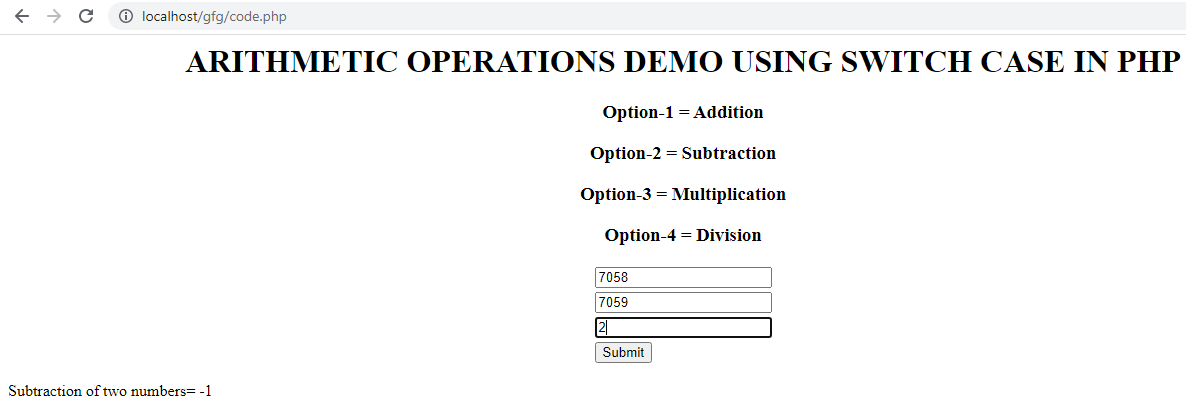Subtraction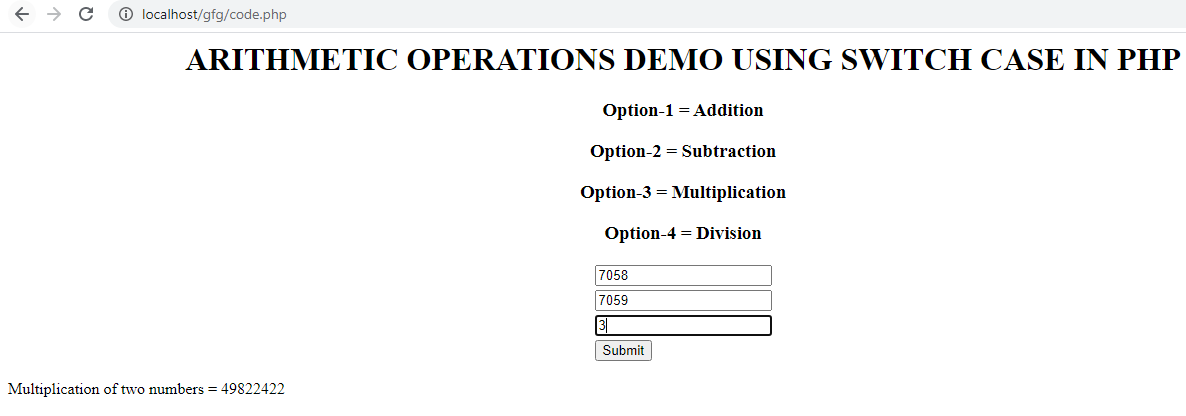Multiplication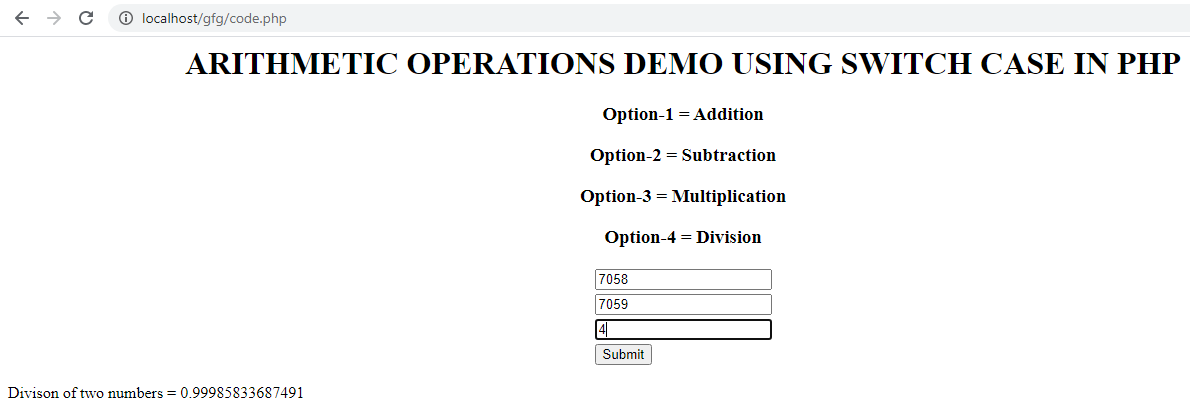Division

My Personal Notes arrow_drop_up